1595726340

# Implementing Logic Gates using Neural Networks (Part 2)

Hello everyone!! Before starting with part 2 of implementing logic gates using Neural networks, you would want to go through part1 first.

From part 1, we had figured out that we have two input neurons or x vector having values as x1 and x2 and 1 being the bias value. The input values, i.e., x1, x2, and 1 is multiplied with their respective weight matrix that is W1, W2, and W0. The corresponding value is then fed to the summation neuron where we have the summed value which is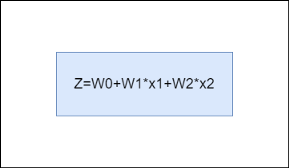Image by Author

Now, this value is fed to a neuron which has a non-linear function(sigmoid in our case) for scaling the output to a desirable range. The scaled output of sigmoid is 0 if the output is less than 0.5 and 1 if the output is greater than 0.5. Our main aim is to find the value of weights or the weight vector which will enable the system to act as a particular gate.

# Implementing AND gate

AND gate operation is a simple multiplication operation between the inputs. If any of the input is 0, the output is 0. In order to achieve 1 as the output, both the inputs should be 1. The truth table below conveys the same information.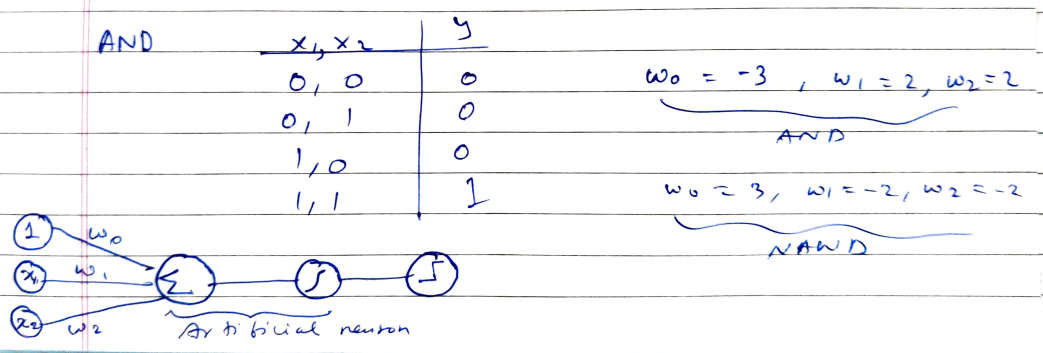Truth Table of AND gate and the values of weights that make the system act as AND and NAND gate, Image by Author

As we have 4 choices of input, the weights must be such that the condition of AND gate is satisfied for all the input points.

# (0,0) case

Consider a situation in which the input or the x vector is (0,0). The value of Z, in that case, will be nothing but W0. Now, W0 will have to be less than 0 so that Z is less than 0.5 and the output or ŷ is 0 and the definition of the AND gate is satisfied. If it is above 0, then the value after Z has passed through the sigmoid function will be 1 which violates the AND gate condition. Hence, we can say with a resolution that W0 has to be a negative value. But what value of W0? Keep reading…

# (0,1) case

Now, consider a situation in which the input or the x vector is (0,1). Here the value of Z will be W0+0+W2*1. This being the input to the sigmoid function should have a value less than 0 so that the output is less than 0.5 and is classified as 0. Henceforth, W0+W2<0. If we take the value of W0 as -3(remember the value of W0 has to be negative) and the value of W2 as +2, the result comes out to be -3+2 and that is -1 which seems to satisfy the above inequality and is at par with the condition of AND gate.

# (1,0) case

Similarly, for the (1,0) case, the value of W0 will be -3 and that of W1 can be +2. Remember you can take any values of the weights W0, W1, and W2 as long as the inequality is preserved.

# (1,1) case

In this case, the input or the x vector is (1,1). The value of Z, in that case, will be nothing but W0+W1+W2. Now, the overall output has to be greater than 0 so that the output is 1 and the definition of the AND gate is satisfied. From previous scenarios, we had found the values of W0, W1, W2 to be -3,2,2 respectively. Placing these values in the Z equation yields an output -3+2+2 which is 1 and greater than 0. This will, therefore, be classified as 1 after passing through the sigmoid function.

# A final note on AND and NAND implementation

The line separating the above four points, therefore, be an equation W0+W1x1+W2x2=0 where W0 is -3, and both W1 and W2 are +2. The equation of the line of separation of four points is therefore x1+x2=3/2. The implementation of the NOR gate will, therefore, be similar to the just the weights being changed to W0 equal to 3, and that of W1 and W2 equal to -2

#deep-learning #deep learning

## Buddha Community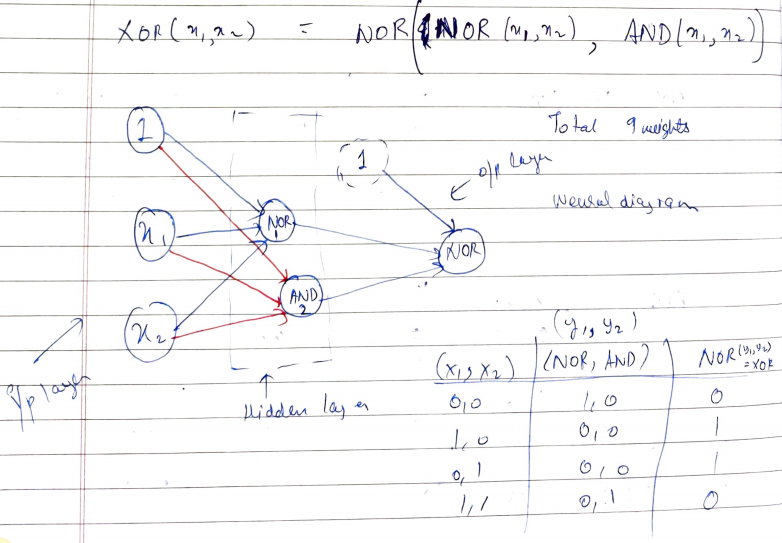1595435940

## Implementing Logic Gates using Neural Networks (Part 1)

Today, I will be discussing the applications of neural networks and how they can be used as logic gates. Logic gates form the basis of any complex calculations that we perform from addition to subtraction to integration and even derivation. Logic gates such as OR, NOR, AND, NAND and XOR are prevalent and a lot of us have come across with their respective truth tables at some point in our life.

# Starting off with Neural Networks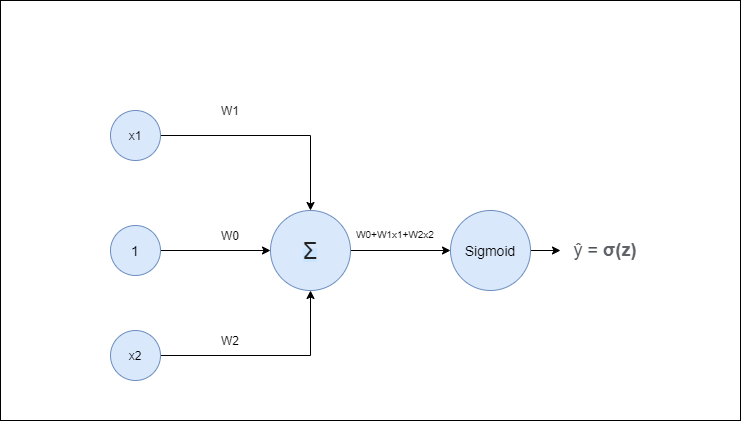Neural Network

Above is the figure of a simple neural network. Here, we have 2 input neurons or x vector having values as x1 and x2. The input neuron with value 1 is for the bias weight. The input values i.e x1, x2, and 1 are multiplied with their respective weight matrix which is W1, W2, and W0 respectively. The corresponding value is then fed to the summation neuron where we have the summed value which is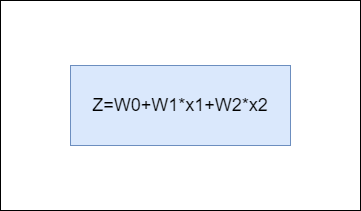Feed to the sigmoid neuron

The value so obtained is fed to a neuron which has a non-linear function(sigmoid in our case) for scaling the output to a desirable range. The output range of sigmoid is 0 if the output is less than 0.5 and 1 if the output is greater than 0.5.

#machine-learning #artificial-intelligence #neural-networks #logic-gates #deep-learning #deep learning1595728140

## Implementing Logic Gates using Neural Networks (Part 2)

Hello everyone!! Before starting with part 2 of implementing logic gates using Neural networks, you would want to go through part1 first.

From part 1, we had figured out that we have two input neurons or x vector having values as x1 and x2 and 1 being the bias value. The input values, i.e., x1, x2, and 1 is multiplied with their respective weight matrix that is W1, W2, and W0. The corresponding value is then fed to the summation neuron where we have the summed value which isNow, this value is fed to a neuron which has a non-linear function(sigmoid in our case) for scaling the output to a desirable range. The scaled output of sigmoid is 0 if the output is less than 0.5 and 1 if the output is greater than 0.5. Our main aim is to find the value of weights or the weight vector which will enable the system to act as a particular gate.

# Implementing AND gate

AND gate operation is a simple multiplication operation between the inputs. If any of the input is 0, the output is 0. In order to achieve 1 as the output, both the inputs should be 1. The truth table below conveys the same information.Truth Table of AND gate and the values of weights that make the system act as AND and NAND gate, Image by Author

As we have 4 choices of input, the weights must be such that the condition of AND gate is satisfied for all the input points.

# (0,0) case

Consider a situation in which the input or the x vector is (0,0). The value of Z, in that case, will be nothing but W0. Now, W0 will have to be less than 0 so that Z is less than 0.5 and the output or ŷ is 0 and the definition of the AND gate is satisfied. If it is above 0, then the value after Z has passed through the sigmoid function will be 1 which violates the AND gate condition. Hence, we can say with a resolution that W0 has to be a negative value. But what value of W0? Keep reading…

# (0,1) case

Now, consider a situation in which the input or the x vector is (0,1). Here the value of Z will be W0+0+W2*1. This being the input to the sigmoid function should have a value less than 0 so that the output is less than 0.5 and is classified as 0. Henceforth, W0+W2<0. If we take the value of W0 as -3(remember the value of W0 has to be negative) and the value of W2 as +2, the result comes out to be -3+2 and that is -1 which seems to satisfy the above inequality and is at par with the condition of AND gate.

# (1,0) case

Similarly, for the (1,0) case, the value of W0 will be -3 and that of W1 can be +2. Remember you can take any values of the weights W0, W1, and W2 as long as the inequality is preserved.

# (1,1) case

In this case, the input or the x vector is (1,1). The value of Z, in that case, will be nothing but W0+W1+W2. Now, the overall output has to be greater than 0 so that the output is 1 and the definition of the AND gate is satisfied. From previous scenarios, we had found the values of W0, W1, W2 to be -3,2,2 respectively. Placing these values in the Z equation yields an output -3+2+2 which is 1 and greater than 0. This will, therefore, be classified as 1 after passing through the sigmoid function.

# A final note on AND and NAND implementation

The line separating the above four points, therefore, be an equation W0+W1x1+W2x2=0 where W0 is -3, and both W1 and W2 are +2. The equation of the line of separation of four points is therefore x1+x2=3/2. The implementation of the NOR gate will, therefore, be similar to the just the weights being changed to W0 equal to 3, and that of W1 and W2 equal to -2

#geometry #logic-gates #deep-learning #deep learning1631544191

## Let Developers Just Need to Grasp only One Button Component

From then on, developers only need to master one Button component, which is enough.

Author：Newton(coorchice.cb@alibaba-inc.com)

✨ Features

Rich corner effect

Exquisite border decoration

Flexible icon support

Cool interaction Special effects

High-quality Neumorphism style

🛠 Guide

## 📺 Demo

### 🔩 Basic Demo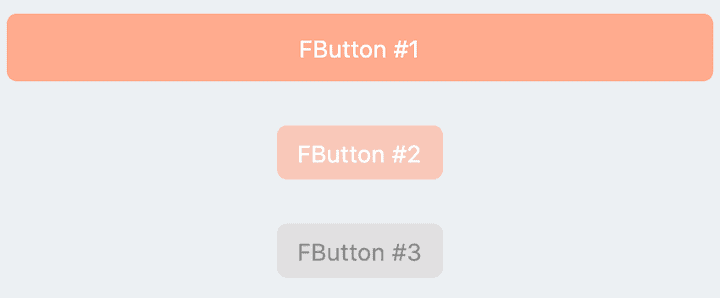``````// FButton #1
FButton(
height: 40,
alignment: Alignment.center,
text: "FButton #1",
style: TextStyle(color: Colors.white),
color: Color(0xffffab91),
onPressed: () {},
)

// FButton #2
FButton(
padding: const EdgeInsets.fromLTRB(12, 8, 12, 8),
text: "FButton #2",
style: TextStyle(color: Colors.white),
color: Color(0xffffab91),
corner: FCorner.all(6.0),
)

// FButton #3
FButton(
padding: const EdgeInsets.fromLTRB(12, 8, 12, 8),
text: "FButton #3",
style: TextStyle(color: Colors.white),
disableStyle: TextStyle(color: Colors.black38),

/// set disable Color
disabledColor: Colors.grey,
corner: FCorner.all(6.0),
)
``````

By simply configuring `text` and`onPressed`, you can construct an available FButton.

If `onPressed` is not set, FButton will be automatically recognized as not unavailable. At this time, ** FButton ** will have a default unavailable status style.

You can also freely configure the style of FButton when it is not available via the `disabledXXX` attribute.

### 🎈 Corner & Stroke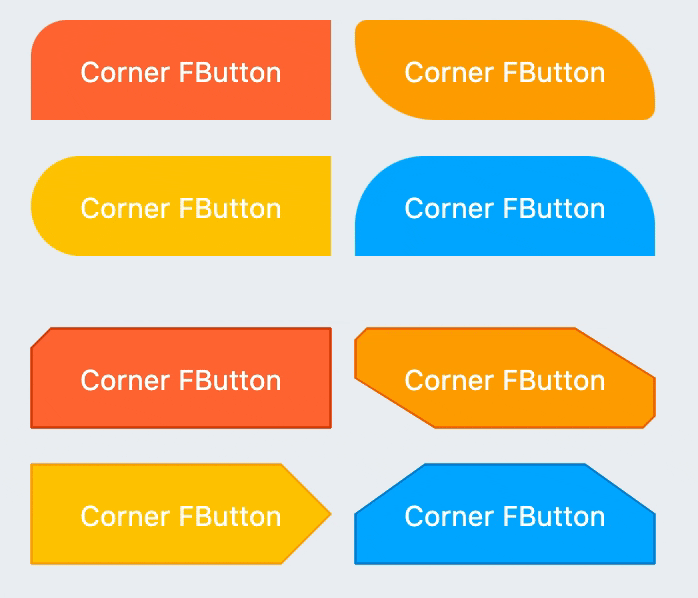``````// #1
FButton(
width: 130,
text: "FButton #1",
style: TextStyle(color: Colors.white),
color: Color(0xffFF7043),
onPressed: () {},
clickEffect: true,

/// 配置边角大小
///
/// set corner size
corner: FCorner.all(25),
),

// #2
FButton(
width: 130,
text: "FButton #2",
style: TextStyle(color: Colors.white),
color: Color(0xffFFA726),
onPressed: () {},
clickEffect: true,
corner: FCorner(
leftBottomCorner: 40,
leftTopCorner: 6,
rightTopCorner: 40,
rightBottomCorner: 6,
),
),

// #3
FButton(
width: 130,
text: "FButton #3",
style: TextStyle(color: Colors.white),
color: Color(0xffFFc900),
onPressed: () {},
clickEffect: true,
corner: FCorner(leftTopCorner: 10),

/// 设置边角风格
///
/// set corner style
cornerStyle: FCornerStyle.bevel,
strokeWidth: 0.5,
strokeColor: Color(0xffF9A825),
),

// #4
FButton(
width: 130,
text: "FButton #4",
style: TextStyle(color: Colors.white),
color: Color(0xff00B0FF),
onPressed: () {},
clickEffect: true,
corner: FCorner(
rightTopCorner: 25,
rightBottomCorner: 25),
cornerStyle: FCornerStyle.bevel,
strokeWidth: 0.5,
strokeColor: Color(0xff000000),
),
``````

You can add rounded corners to FButton via the `corner` property. You can even control each fillet individually。

By default, the corners of FButton are rounded. By setting `cornerStyle: FCornerStyle.bevel`, you can get a bevel effect.

FButton supports control borders, provided that `strokeWidth> 0` can get the effect 🥳.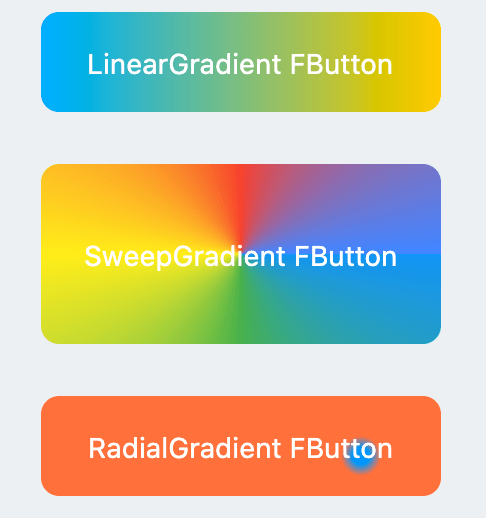``````
FButton(
width: 100,
height: 60,
text: "#1",
style: TextStyle(color: Colors.white),
color: Color(0xffFFc900),

/// 配置渐变色
///
Color(0xff00B0FF),
Color(0xffFFc900),
]),
onPressed: () {},
clickEffect: true,
corner: FCorner.all(8),
)
``````

Through the `gradient` attribute, you can build FButton with gradient colors. You can freely build many types of gradient colors.

### 🍭 Icon``````FButton(
width: 88,
height: 38,
text: "Back",
style: TextStyle(color: Colors.white),
color: Color(0xffffc900),
onPressed: () {
toast(context, "Back!");
},
clickEffect: true,
corner: FCorner(
leftTopCorner: 25,
leftBottomCorner: 25,),

/// 配置图标
///
/// set icon
image: Icon(
Icons.arrow_back_ios,
color: Colors.white,
size: 12,
),

/// 配置图标与文字的间距
///
/// Configure the spacing between icon and text
imageMargin: 8,
),

FButton(
onPressed: () {},
image: Icon(
Icons.print,
color: Colors.grey,
),
imageMargin: 8,

/// 配置图标与文字相对位置
///
/// Configure the relative position of icons and text
imageAlignment: ImageAlignment.top,
text: "Print",
style: TextStyle(color: textColor),
color: Colors.transparent,
),
``````

The `image` property can set an image for FButton and you can adjust the position of the image relative to the text, through`imageAlignment`.

If the button does not need a background, just set `color: Colors.transparent`.

### 🔥 Effect``````
FButton(
width: 200,
text: "Try Me!",
style: TextStyle(color: textColor),
color: Color(0xffffc900),
onPressed: () {},
clickEffect: true,
corner: FCorner.all(9),

/// 配置按下时颜色
///
/// set pressed color
highlightColor: Color(0xffE65100).withOpacity(0.20),

/// 配置 hover 状态时颜色
///
/// set hover color
hoverColor: Colors.redAccent.withOpacity(0.16),
),
``````

The highlight color of FButton can be configured through the `highlightColor` property。

`hoverColor` can configure the color when the mouse moves to the range of FButton, which will be used during Web development.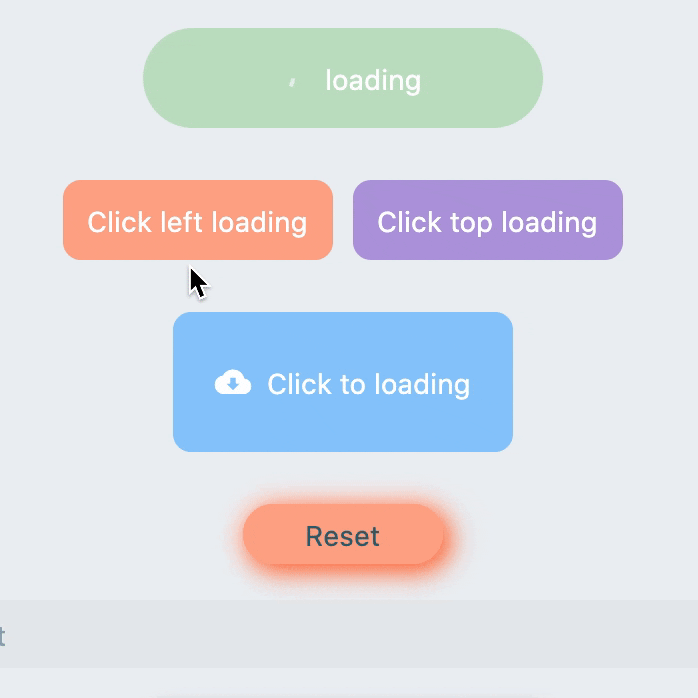``````FButton(
style: TextStyle(color: textColor),
color: Color(0xffffc900),
...

///

///
imageMargin: 6,

///

///

///

///

///
imageAlignment: ImageAlignment.top,
),

// #2
FButton(
width: 170,
height: 70,
style: TextStyle(color: textColor),
color: Color(0xffffc900),
onPressed: () { },
...
imageMargin: 8,

///
)

FButton(
width: 170,
height: 70,
alignment: Alignment.center,
style: TextStyle(color: Colors.white),
color: Color(0xff90caf9),
...
imageMargin: 8,

///
),
``````

Through the `loading` attribute, you can configure Loading effects for ** FButton **.

When FButton is in Loading state, FButton will enter an unavailable state, onPress will no longer be triggered, and unavailable styles will also be applied.

At the same time `loadingText` will overwrite`text` if it is not null.

The click start Loading effect can be achieved through the `clickLoading` attribute.

The position of `loading` will be affected by the`imageAlignment` attribute.

When `hideTextOnLoading: true`, if FButton is in`loading` state, its text will be hidden.

Through `loadingWidget`, developers can set completely customized loading styles.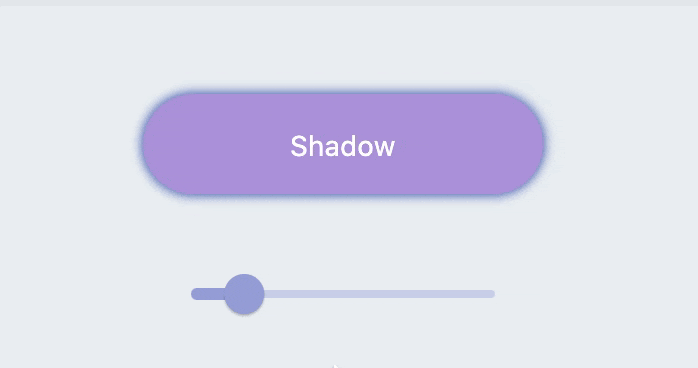``````
FButton(
width: 200,
textColor: Colors.white,
color: Color(0xffffc900),
onPressed: () {},
clickEffect: true,
corner: FCorner.all(28),

/// 配置阴影颜色
///

/// 设置组件高斯与阴影形状卷积的标准偏差。
///
/// Sets the standard deviation of the component's Gaussian convolution with the shadow shape.
),
``````

FButton allows you to configure the color, size, and position of the shadow.

### 🍭 Neumorphism Style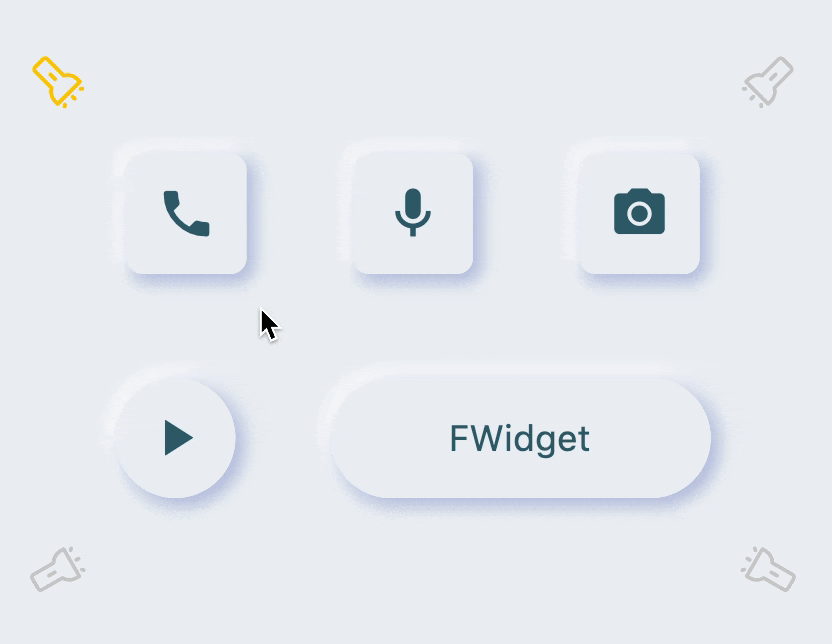``````FButton(

/// 开启 Neumorphism 支持
///
/// Turn on Neumorphism support
isSupportNeumorphism: true,

/// 配置光源方向
///
/// Configure light source direction
lightOrientation: lightOrientation,

/// 配置亮部阴影
///

/// 配置暗部阴影
///
strokeColor: mainBackgroundColor,
strokeWidth: 3.0,
width: 190,
height: 60,
text: "FWidget",
style: TextStyle(
color: mainTextTitleColor, fontSize: neumorphismSize_2_2),
alignment: Alignment.center,
color: mainBackgroundColor,
...
)
``````

FButton brings an incredible, ultra-high texture Neumorphism style to developers.

Developers only need to configure the `isSupportNeumorphism` parameter to enable and disable the Neumorphism style.

If you want to adjust the style of Neumorphism, you can make subtle adjustments through several attributes related to Shadow, among which:

FButton also provides `lightOrientation` parameters, and even allows developers to adjust the care angle, and has obtained different Neumorphism effects.

😃 How to use？

Add dependencies in the project `pubspec.yaml` file:

## 🌐 pub dependency

``````dependencies:
fbutton: ^<version number>
``````

## 🖥 git dependencies

``````dependencies:
fbutton:
git:
url: 'git@github.com:Fliggy-Mobile/fbutton.git'
ref: '<Branch number or tag number>'
``````

## Use this package as a library

### Depend on it

Run this command:

With Flutter:

`` \$ flutter pub add fbutton_nullsafety``

This will add a line like this to your package's pubspec.yaml (and run an implicit `flutter pub get`):

``````dependencies:
fbutton_nullsafety: ^5.0.0``````

Alternatively, your editor might support or `flutter pub get`. Check the docs for your editor to learn more.

### Import it

Now in your Dart code, you can use:

``import 'package:fbutton_nullsafety/fbutton_nullsafety.dart';``

Author: Fliggy-Mobile

Source Code: https://github.com/Fliggy-Mobile/fbutton1596825840

## A Comparative Analysis of Recurrent Neural Networks

Recurrent neural networks, also known as RNNs, are a class of neural networks that allow previous outputs to be used as inputs while having hidden states. RNN models are mostly used in the fields of natural language processing and speech recognition.

The vanishing and exploding gradient phenomena are often encountered in the context of RNNs. The reason why they happen is that it is difficult to capture long term dependencies because of multiplicative gradient that can be exponentially decreasing/increasing with respect to the number of layers.

Gated Recurrent Unit (GRU) and Long Short-Term Memory units (LSTM) deal with the vanishing gradient problem encountered by traditional RNNs, with LSTM being a generalization of GRU.

1D Convolution_ layer_ creates a convolution kernel that is convolved with the layer input over a single spatial (or temporal) dimension to produce a tensor of outputs. It is very effective for deriving features from a fixed-length segment of the overall dataset. A 1D CNN works well for natural language processing (NLP).

## DATASET: IMDb Movie Review

TensorFlow Datasets is a collection of datasets ready to use, with TensorFlow or other Python ML frameworks, such as Jax. All datasets are exposed as `[_tf.data.Datasets_](https://www.tensorflow.org/api_docs/python/tf/data/Dataset)`, enabling easy-to-use and high-performance input pipelines.

“imdb_reviews”

This is a dataset for binary sentiment classification containing substantially more data than previous benchmark datasets. It provides a set of 25,000 highly polar movie reviews for training, and 25,000 for testing.

## Import Libraries

``````import pandas as pd
import numpy as np
import matplotlib.pyplot as plt
import seaborn as sns
%matplotlib inline
``````

``````import tensorflow as tf
import tensorflow_datasets

imdb
``````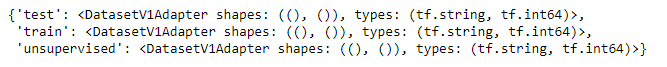``````info
``````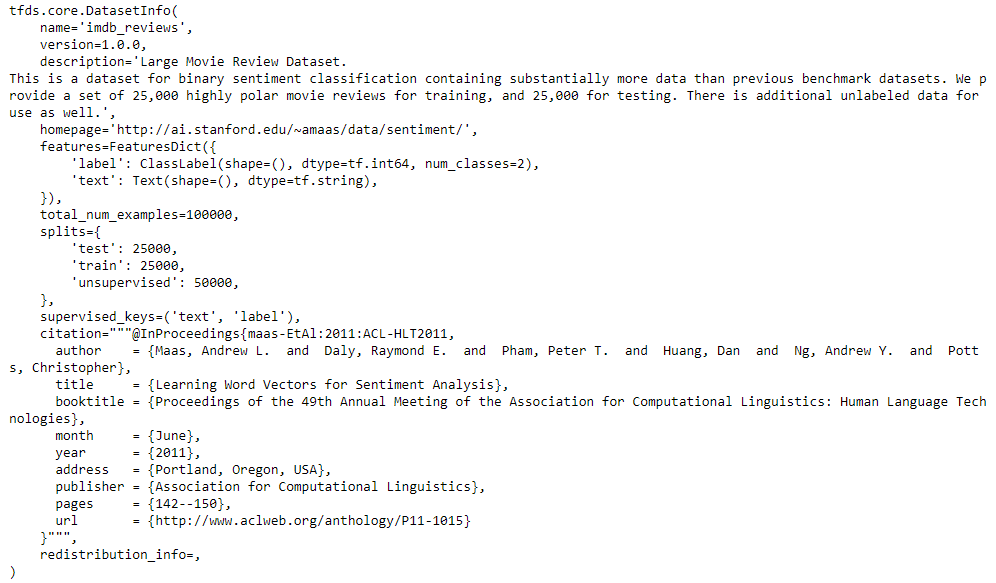## Training and Testing Data

``````train_data, test_data=imdb['train'], imdb['test']

training_sentences=[]
training_label=[]
testing_sentences=[]
testing_label=[]
for s,l in train_data:
training_sentences.append(str(s.numpy()))
training_label.append(l.numpy())
for s,l in test_data:
testing_sentences.append(str(s.numpy()))
testing_label.append(l.numpy())
training_label_final=np.array(training_label)
testing_label_final=np.array(testing_label)
``````

``````vocab_size=10000
embedding_dim=16
max_length=120
trunc_type='post'
oov_tok='<oov>'
from tensorflow.keras.preprocessing.text import Tokenizer
tokenizer= Tokenizer(num_words=vocab_size, oov_token=oov_tok)
tokenizer.fit_on_texts(training_sentences)
word_index=tokenizer.word_index
sequences=tokenizer.texts_to_sequences(training_sentences)
testing_sequences=tokenizer.texts_to_sequences(testing_sentences)
from tensorflow.keras.models import Sequential
from tensorflow.keras.layers import Dense, Dropout, Embedding
``````

## Multi-layer Bidirectional LSTM

#imdb #convolutional-network #long-short-term-memory #recurrent-neural-network #gated-recurrent-unit #neural networks1623135499

## No Code introduction to Neural Networks

### The simple architecture explained

Neural networks have been around for a long time, being developed in the 1960s as a way to simulate neural activity for the development of artificial intelligence systems. However, since then they have developed into a useful analytical tool often used in replace of, or in conjunction with, standard statistical models such as regression or classification as they can be used to predict or more a specific output. The main difference, and advantage, in this regard is that neural networks make no initial assumptions as to the form of the relationship or distribution that underlies the data, meaning they can be more flexible and capture non-standard and non-linear relationships between input and output variables, making them incredibly valuable in todays data rich environment.

In this sense, their use has took over the past decade or so, with the fall in costs and increase in ability of general computing power, the rise of large datasets allowing these models to be trained, and the development of frameworks such as TensforFlow and Keras that have allowed people with sufficient hardware (in some cases this is no longer even an requirement through cloud computing), the correct data and an understanding of a given coding language to implement them. This article therefore seeks to be provide a no code introduction to their architecture and how they work so that their implementation and benefits can be better understood.

Firstly, the way these models work is that there is an input layer, one or more hidden layers and an output layer, each of which are connected by layers of synaptic weights¹. The input layer (X) is used to take in scaled values of the input, usually within a standardised range of 0–1. The hidden layers (Z) are then used to define the relationship between the input and output using weights and activation functions. The output layer (Y) then transforms the results from the hidden layers into the predicted values, often also scaled to be within 0–1. The synaptic weights (W) connecting these layers are used in model training to determine the weights assigned to each input and prediction in order to get the best model fit. Visually, this is represented as:

#machine-learning #python #neural-networks #tensorflow #neural-network-algorithm #no code introduction to neural networks